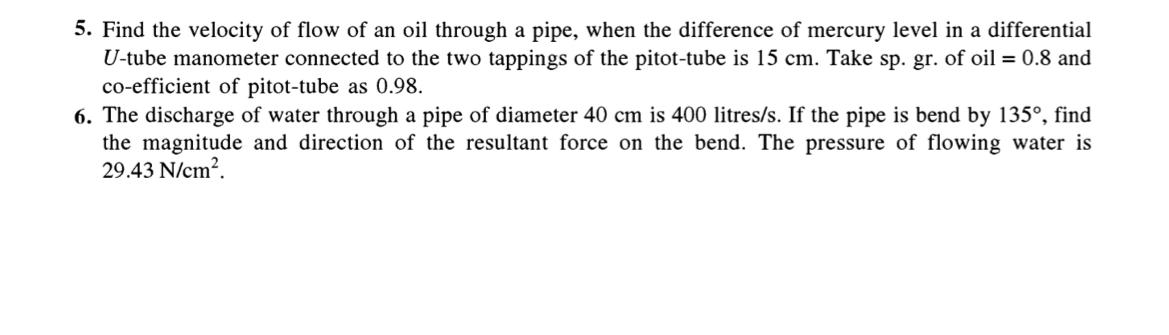# Q5. Find the velocity of flow of an oil through a pipe, when the difference of mercury level in a differential U-tube manometer connected to the two tappings of the pitot-tube is 15 cm. Take sp. gr. of oil = 0.8 and co-efficient of pitot-tube as 0.98.  Q6. The discharge of water through a pipe of diameter 40 cm is 400 litres/s. If the pipe is bend by 135°, find the magnitude and direction of the resultant force on the bend. The pressure of flowing water is 29.43 N/cm²

Question-AnswerCategory: Fluid MechanicsQ5. Find the velocity of flow of an oil through a pipe, when the difference of mercury level in a differential U-tube manometer connected to the two tappings of the pitot-tube is 15 cm. Take sp. gr. of oil = 0.8 and co-efficient of pitot-tube as 0.98.  Q6. The discharge of water through a pipe of diameter 40 cm is 400 litres/s. If the pipe is bend by 135°, find the magnitude and direction of the resultant force on the bend. The pressure of flowing water is 29.43 N/cm²

Q5. Find the velocity of flow of an oil through a pipe, when the difference of mercury level in a differential U-tube manometer connected to the two tappings of the pitot-tube is 15 cm. Take sp. gr. of oil = 0.8 and co-efficient of pitot-tube as 0.98.
Q6. The discharge of water through a pipe of diameter 40 cm is 400 litres/s. If the pipe is bend by 135°, find the magnitude and direction of the resultant force on the bend. The pressure of flowing water is 29.43 N/cm².